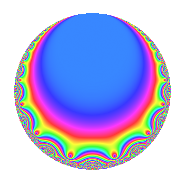# Properties

 Label 8001.2.a.kLevel 8001 Weight 2 Character orbit 8001.a Self dual Yes Analytic conductor 63.888 Analytic rank 0 Dimension 2 CM No Inner twists 1

# Related objects

## Newspace parameters

 Level: $$N$$ = $$8001 = 3^{2} \cdot 7 \cdot 127$$ Weight: $$k$$ = $$2$$ Character orbit: $$[\chi]$$ = 8001.a (trivial)

## Newform invariants

 Self dual: Yes Analytic conductor: $$63.8883066572$$ Analytic rank: $$0$$ Dimension: $$2$$ Coefficient field: $$\Q(\sqrt{6})$$ Coefficient ring: $$\Z[a_1, a_2]$$ Coefficient ring index: $$1$$ Fricke sign: $$-1$$ Sato-Tate group: $\mathrm{SU}(2)$

## $q$-expansion

Coefficients of the $$q$$-expansion are expressed in terms of $$\beta = \sqrt{6}$$. We also show the integral $$q$$-expansion of the trace form.

 $$f(q)$$ $$=$$ $$q$$ $$+ \beta q^{2}$$ $$+ 4 q^{4}$$ $$+ \beta q^{5}$$ $$+ q^{7}$$ $$+ 2 \beta q^{8}$$ $$+O(q^{10})$$ $$q$$ $$+ \beta q^{2}$$ $$+ 4 q^{4}$$ $$+ \beta q^{5}$$ $$+ q^{7}$$ $$+ 2 \beta q^{8}$$ $$+ 6 q^{10}$$ $$+ ( 2 + 2 \beta ) q^{13}$$ $$+ \beta q^{14}$$ $$+ 4 q^{16}$$ $$+ ( 3 - \beta ) q^{17}$$ $$+ ( 2 - \beta ) q^{19}$$ $$+ 4 \beta q^{20}$$ $$-6 q^{23}$$ $$+ q^{25}$$ $$+ ( 12 + 2 \beta ) q^{26}$$ $$+ 4 q^{28}$$ $$+ ( 3 - 2 \beta ) q^{29}$$ $$+ 8 q^{31}$$ $$+ ( -6 + 3 \beta ) q^{34}$$ $$+ \beta q^{35}$$ $$-7 q^{37}$$ $$+ ( -6 + 2 \beta ) q^{38}$$ $$+ 12 q^{40}$$ $$+ ( -3 + \beta ) q^{41}$$ $$+ 2 q^{43}$$ $$-6 \beta q^{46}$$ $$+ 12 q^{47}$$ $$+ q^{49}$$ $$+ \beta q^{50}$$ $$+ ( 8 + 8 \beta ) q^{52}$$ $$+ ( 3 + 2 \beta ) q^{53}$$ $$+ 2 \beta q^{56}$$ $$+ ( -12 + 3 \beta ) q^{58}$$ $$-\beta q^{59}$$ $$+ ( 2 - \beta ) q^{61}$$ $$+ 8 \beta q^{62}$$ $$-8 q^{64}$$ $$+ ( 12 + 2 \beta ) q^{65}$$ $$+ ( 2 + 5 \beta ) q^{67}$$ $$+ ( 12 - 4 \beta ) q^{68}$$ $$+ 6 q^{70}$$ $$+ ( -6 - \beta ) q^{71}$$ $$+ ( -4 - 3 \beta ) q^{73}$$ $$-7 \beta q^{74}$$ $$+ ( 8 - 4 \beta ) q^{76}$$ $$+ ( -1 - 2 \beta ) q^{79}$$ $$+ 4 \beta q^{80}$$ $$+ ( 6 - 3 \beta ) q^{82}$$ $$-6 q^{83}$$ $$+ ( -6 + 3 \beta ) q^{85}$$ $$+ 2 \beta q^{86}$$ $$-4 \beta q^{89}$$ $$+ ( 2 + 2 \beta ) q^{91}$$ $$-24 q^{92}$$ $$+ 12 \beta q^{94}$$ $$+ ( -6 + 2 \beta ) q^{95}$$ $$+ ( 5 + 3 \beta ) q^{97}$$ $$+ \beta q^{98}$$ $$+O(q^{100})$$ $$\operatorname{Tr}(f)(q)$$ $$=$$ $$2q$$ $$\mathstrut +\mathstrut 8q^{4}$$ $$\mathstrut +\mathstrut 2q^{7}$$ $$\mathstrut +\mathstrut O(q^{10})$$ $$2q$$ $$\mathstrut +\mathstrut 8q^{4}$$ $$\mathstrut +\mathstrut 2q^{7}$$ $$\mathstrut +\mathstrut 12q^{10}$$ $$\mathstrut +\mathstrut 4q^{13}$$ $$\mathstrut +\mathstrut 8q^{16}$$ $$\mathstrut +\mathstrut 6q^{17}$$ $$\mathstrut +\mathstrut 4q^{19}$$ $$\mathstrut -\mathstrut 12q^{23}$$ $$\mathstrut +\mathstrut 2q^{25}$$ $$\mathstrut +\mathstrut 24q^{26}$$ $$\mathstrut +\mathstrut 8q^{28}$$ $$\mathstrut +\mathstrut 6q^{29}$$ $$\mathstrut +\mathstrut 16q^{31}$$ $$\mathstrut -\mathstrut 12q^{34}$$ $$\mathstrut -\mathstrut 14q^{37}$$ $$\mathstrut -\mathstrut 12q^{38}$$ $$\mathstrut +\mathstrut 24q^{40}$$ $$\mathstrut -\mathstrut 6q^{41}$$ $$\mathstrut +\mathstrut 4q^{43}$$ $$\mathstrut +\mathstrut 24q^{47}$$ $$\mathstrut +\mathstrut 2q^{49}$$ $$\mathstrut +\mathstrut 16q^{52}$$ $$\mathstrut +\mathstrut 6q^{53}$$ $$\mathstrut -\mathstrut 24q^{58}$$ $$\mathstrut +\mathstrut 4q^{61}$$ $$\mathstrut -\mathstrut 16q^{64}$$ $$\mathstrut +\mathstrut 24q^{65}$$ $$\mathstrut +\mathstrut 4q^{67}$$ $$\mathstrut +\mathstrut 24q^{68}$$ $$\mathstrut +\mathstrut 12q^{70}$$ $$\mathstrut -\mathstrut 12q^{71}$$ $$\mathstrut -\mathstrut 8q^{73}$$ $$\mathstrut +\mathstrut 16q^{76}$$ $$\mathstrut -\mathstrut 2q^{79}$$ $$\mathstrut +\mathstrut 12q^{82}$$ $$\mathstrut -\mathstrut 12q^{83}$$ $$\mathstrut -\mathstrut 12q^{85}$$ $$\mathstrut +\mathstrut 4q^{91}$$ $$\mathstrut -\mathstrut 48q^{92}$$ $$\mathstrut -\mathstrut 12q^{95}$$ $$\mathstrut +\mathstrut 10q^{97}$$ $$\mathstrut +\mathstrut O(q^{100})$$

## Embeddings

For each embedding $$\iota_m$$ of the coefficient field, the values $$\iota_m(a_n)$$ are shown below.

For more information on an embedded modular form you can click on its label.

Label $$\iota_m(\nu)$$ $$a_{2}$$ $$a_{3}$$ $$a_{4}$$ $$a_{5}$$ $$a_{6}$$ $$a_{7}$$ $$a_{8}$$ $$a_{9}$$ $$a_{10}$$
1.1
 −2.44949 2.44949
−2.44949 0 4.00000 −2.44949 0 1.00000 −4.89898 0 6.00000
1.2 2.44949 0 4.00000 2.44949 0 1.00000 4.89898 0 6.00000
 $$n$$: e.g. 2-40 or 990-1000 Significant digits: Format: Complex embeddings Normalized embeddings Satake parameters Satake angles

## Inner twists

This newform does not admit any (nontrivial) inner twists.

## Atkin-Lehner signs

$$p$$ Sign
$$3$$ $$-1$$
$$7$$ $$-1$$
$$127$$ $$-1$$

## Hecke kernels

This newform can be constructed as the intersection of the kernels of the following linear operators acting on $$S_{2}^{\mathrm{new}}(\Gamma_0(8001))$$:

 $$T_{2}^{2}$$ $$\mathstrut -\mathstrut 6$$ $$T_{5}^{2}$$ $$\mathstrut -\mathstrut 6$$